oomph::ComplexGMRES< MATRIX > Class Template Reference

The GMRES method rewritten for complex matrices. More...

#include <complex_smoother.h>Inheritance diagram for oomph::ComplexGMRES< MATRIX >:

## Public Member Functions

ComplexGMRES ()
Constructor. More...

~ComplexGMRES ()
Empty destructor. More...

ComplexGMRES (const ComplexGMRES &)=delete
Broken copy constructor. More...

void operator= (const ComplexGMRES &)=delete
Broken assignment operator. More...

void disable_resolve ()
Overload disable resolve so that it cleans up memory too. More...

void solve (Problem *const &problem_pt, DoubleVector &result)
Solver: Takes pointer to problem and returns the results vector which contains the solution of the linear system defined by the problem's fully assembled Jacobian and residual vector. More...

void solve (DoubleMatrixBase *const &matrix_pt, const Vector< double > &rhs, Vector< double > &result)
Linear-algebra-type solver: Takes pointer to a matrix and rhs vector and returns the solution of the linear system Call the broken base-class version. If you want this, please implement it. More...

unsigned iterations () const
Number of iterations taken. More...

void complex_smoother_setup (Vector< CRDoubleMatrix * > helmholtz_matrix_pt)
Setup: Pass pointer to the matrix and store in cast form. More...

void complex_smoother_solve (const Vector< DoubleVector > &rhs, Vector< DoubleVector > &solution)
The smoother_solve function performs fixed number of iterations on the system A*result=rhs. The number of (smoothing) iterations is the same as the max. number of iterations in the underlying IterativeLinearSolver class. More...Public Member Functions inherited from oomph::HelmholtzSmoother
HelmholtzSmoother ()
Empty constructor. More...

virtual ~HelmholtzSmoother ()
Virtual empty destructor. More...

void complex_matrix_multiplication (Vector< CRDoubleMatrix * > matrices_pt, const Vector< DoubleVector > &x, Vector< DoubleVector > &soln)
Helper function to calculate a complex matrix-vector product. Assumes the matrix has been provided as a Vector of length two; the first entry containing the real part of the system matrix and the second entry containing the imaginary part. More...

template<typename MATRIX >
void check_validity_of_solve_helper_inputs (CRDoubleMatrix *const &real_matrix_pt, CRDoubleMatrix *const &imag_matrix_pt, const Vector< DoubleVector > &rhs, Vector< DoubleVector > &solution, const double &n_dof)
Self-test to check that all the dimensions of the inputs to solve helper are consistent and everything that needs to be built, is. More...Public Member Functions inherited from oomph::IterativeLinearSolver
IterativeLinearSolver ()
Constructor: Set (default) trivial preconditioner and set defaults for tolerance and max. number of iterations. More...

IterativeLinearSolver (const IterativeLinearSolver &)=delete
Broken copy constructor. More...

void operator= (const IterativeLinearSolver &)=delete
Broken assignment operator. More...

virtual ~IterativeLinearSolver ()
Destructor (empty) More...

Preconditioner *& preconditioner_pt ()
Access function to preconditioner. More...

Preconditioner *const & preconditioner_pt () const
Access function to preconditioner (const version) More...

double & tolerance ()

unsigned & max_iter ()

void enable_doc_convergence_history ()
Enable documentation of the convergence history. More...

void disable_doc_convergence_history ()
Disable documentation of the convergence history. More...

void open_convergence_history_file_stream (const std::string &file_name, const std::string &zone_title="")
Write convergence history into file with specified filename (automatically switches on doc). Optional second argument is a string that can be used (as a zone title) to identify what case we're running (e.g. what combination of linear solver and preconditioner or parameter values are used). More...

void close_convergence_history_file_stream ()
Close convergence history output stream. More...

double jacobian_setup_time () const
returns the time taken to assemble the jacobian matrix and residual vector More...

double linear_solver_solution_time () const
return the time taken to solve the linear system More...

virtual double preconditioner_setup_time () const
returns the the time taken to setup the preconditioner More...

void enable_setup_preconditioner_before_solve ()
Setup the preconditioner before the solve. More...

void disable_setup_preconditioner_before_solve ()
Don't set up the preconditioner before the solve. More...

void enable_error_after_max_iter ()
Throw an error if we don't converge within max_iter. More...

void disable_error_after_max_iter ()
Don't throw an error if we don't converge within max_iter (default). More...

void enable_iterative_solver_as_preconditioner ()
Enables the iterative solver be used as preconditioner (when calling the solve method it bypass the setup solver method – currently only used by Trilinos solver —) More...

void disable_iterative_solver_as_preconditioner ()
Disables the iterative solver be used as preconditioner (when calling the solve method it bypass the setup solver method – currently only used by Trilinos solver —) More...Public Member Functions inherited from oomph::LinearSolver
LinearSolver ()
Empty constructor, initialise the member data. More...

LinearSolver (const LinearSolver &dummy)=delete
Broken copy constructor. More...

void operator= (const LinearSolver &)=delete
Broken assignment operator. More...

virtual ~LinearSolver ()
Empty virtual destructor. More...

void enable_doc_time ()
Enable documentation of solve times. More...

void disable_doc_time ()
Disable documentation of solve times. More...

bool is_doc_time_enabled () const
Is documentation of solve times enabled? More...

bool is_resolve_enabled () const
Boolean flag indicating if resolves are enabled. More...

virtual void enable_resolve ()
Enable resolve (i.e. store matrix and/or LU decomposition, say) Virtual so it can be overloaded to perform additional tasks. More...

virtual void solve (DoubleMatrixBase *const &matrix_pt, const DoubleVector &rhs, DoubleVector &result)
Linear-algebra-type solver: Takes pointer to a matrix and rhs vector and returns the solution of the linear system. More...

virtual void solve_transpose (Problem *const &problem_pt, DoubleVector &result)
Solver: Takes pointer to problem and returns the results vector which contains the solution of the linear system defined by the problem's fully assembled Jacobian and residual vector (broken virtual). More...

virtual void solve_transpose (DoubleMatrixBase *const &matrix_pt, const DoubleVector &rhs, DoubleVector &result)
Linear-algebra-type solver: Takes pointer to a matrix and rhs vector and returns the solution of the linear system. More...

virtual void solve_transpose (DoubleMatrixBase *const &matrix_pt, const Vector< double > &rhs, Vector< double > &result)
Linear-algebra-type solver: Takes pointer to a matrix and rhs vector and returns the solution of the linear system. More...

virtual void resolve (const DoubleVector &rhs, DoubleVector &result)
Resolve the system defined by the last assembled jacobian and the rhs vector. Solution is returned in the vector result. (broken virtual) More...

virtual void resolve_transpose (const DoubleVector &rhs, DoubleVector &result)
Solver: Resolve the system defined by the last assembled jacobian and the rhs vector. Solution is returned in the vector result. (broken virtual) More...

function to enable the computation of the gradient required for the globally convergent Newton method More...

function to disable the computation of the gradient required for the globally convergent Newton method More...

function to reset the size of the gradient before each Newton solve More...

function to access the gradient, provided it has been computed More...Public Member Functions inherited from oomph::DistributableLinearAlgebraObject
DistributableLinearAlgebraObject ()
Default constructor - create a distribution. More...

DistributableLinearAlgebraObject (const DistributableLinearAlgebraObject &matrix)=delete
Broken copy constructor. More...

void operator= (const DistributableLinearAlgebraObject &)=delete
Broken assignment operator. More...

virtual ~DistributableLinearAlgebraObject ()
Destructor. More...

unsigned nrow () const
access function to the number of global rows. More...

unsigned nrow_local () const
access function for the num of local rows on this processor. More...

unsigned nrow_local (const unsigned &p) const
access function for the num of local rows on this processor. More...

unsigned first_row () const
access function for the first row on this processor More...

unsigned first_row (const unsigned &p) const
access function for the first row on this processor More...

bool distributed () const
distribution is serial or distributed More...

bool distribution_built () const
if the communicator_pt is null then the distribution is not setup then false is returned, otherwise return true More...

void build_distribution (const LinearAlgebraDistribution *const dist_pt)
setup the distribution of this distributable linear algebra object More...

setup the distribution of this distributable linear algebra object More...

## Private Member Functions

void clean_up_memory ()
Cleanup data that's stored for resolve (if any has been stored) More...

void complex_solve_helper (const Vector< DoubleVector > &rhs, Vector< DoubleVector > &solution)
This is where the actual work is done. More...

void update (const unsigned &k, const Vector< Vector< std::complex< double > > > &hessenberg, const Vector< std::complex< double > > &s, const Vector< Vector< DoubleVector > > &v, Vector< DoubleVector > &x)
Helper function to update the result vector. More...

void generate_plane_rotation (std::complex< double > &dx, std::complex< double > &dy, std::complex< double > &cs, std::complex< double > &sn)
Helper function: Generate a plane rotation. This is done by finding the value of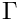(i.e. cs) and the value of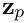(i.e. sn) such that: More...

void apply_plane_rotation (std::complex< double > &dx, std::complex< double > &dy, std::complex< double > &cs, std::complex< double > &sn)
Helper function: Apply plane rotation. This is done using the update: More...

## Private Attributes

unsigned Iterations
Number of iterations taken. More...

Vector< CRDoubleMatrix * > Matrices_storage_pt
Vector of pointers to the real and imaginary part of the system matrix. More...

bool Resolving
Boolean flag to indicate if the solve is done in re-solve mode, bypassing setup of matrix and preconditioner. More...

bool Matrix_can_be_deleted
Boolean flag to indicate if the real and imaginary system matrices can be deleted. More...Protected Member Functions inherited from oomph::DistributableLinearAlgebraObject
void clear_distribution ()
clear the distribution of this distributable linear algebra object More...Protected Attributes inherited from oomph::HelmholtzSmoother
bool Use_as_smoother
When a derived class object is being used as a smoother in the MG algorithm the residual norm does not need to be calculated. This boolean is used as a flag to indicate this in solve_helper(...) More...Protected Attributes inherited from oomph::IterativeLinearSolver
bool Doc_convergence_history
Flag indicating if the convergence history is to be documented. More...

std::ofstream Output_file_stream
Output file stream for convergence history. More...

double Tolerance
Convergence tolerance. More...

unsigned Max_iter
Maximum number of iterations. More...

PreconditionerPreconditioner_pt
Pointer to the preconditioner. More...

double Jacobian_setup_time
Jacobian setup time. More...

double Solution_time
linear solver solution time More...

double Preconditioner_setup_time
Preconditioner setup time. More...

bool Setup_preconditioner_before_solve
indicates whether the preconditioner should be setup before solve. Default = true; More...

bool Throw_error_after_max_iter
Should we throw an error instead of just returning when we hit the max iterations? More...

bool Use_iterative_solver_as_preconditioner
Use the iterative solver as preconditioner. More...

bool First_time_solve_when_used_as_preconditioner
When the iterative solver is used a preconditioner then we call the setup of solver method only once (the first time the solve method is called) More...Protected Attributes inherited from oomph::LinearSolver
bool Enable_resolve
Boolean that indicates whether the matrix (or its factors, in the case of direct solver) should be stored so that the resolve function can be used. More...

bool Doc_time
Boolean flag that indicates whether the time taken. More...

flag that indicates whether the gradient required for the globally convergent Newton method should be computed or not More...

flag that indicates whether the gradient was computed or not More...

DoubleVector storing the gradient for the globally convergent Newton method. More...Static Protected Attributes inherited from oomph::IterativeLinearSolver
static IdentityPreconditioner Default_preconditioner
Default preconditioner: The base class for preconditioners is a fully functional (if trivial!) preconditioner. More...

## Detailed Description

template<typename MATRIX>
class oomph::ComplexGMRES< MATRIX >

The GMRES method rewritten for complex matrices.

Definition at line 854 of file complex_smoother.h.

## ◆ ComplexGMRES() [1/2]

template<typename MATRIX >
 oomph::ComplexGMRES< MATRIX >::ComplexGMRES ( )
inline

Constructor.

Definition at line 858 of file complex_smoother.h.

## ◆ ~ComplexGMRES()

template<typename MATRIX >
 oomph::ComplexGMRES< MATRIX >::~ComplexGMRES ( )
inline

Empty destructor.

Definition at line 867 of file complex_smoother.h.

## ◆ ComplexGMRES() [2/2]

template<typename MATRIX >
 oomph::ComplexGMRES< MATRIX >::ComplexGMRES ( const ComplexGMRES< MATRIX > & )
delete

Broken copy constructor.

## ◆ apply_plane_rotation()

template<typename MATRIX >
 void oomph::ComplexGMRES< MATRIX >::apply_plane_rotation ( std::complex< double > & dx, std::complex< double > & dy, std::complex< double > & cs, std::complex< double > & sn )
inlineprivate

Helper function: Apply plane rotation. This is done using the update: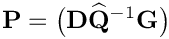Taken from: Saad Y."Iterative methods for sparse linear systems", p.193.

Definition at line 1185 of file complex_smoother.h.

## ◆ clean_up_memory()

template<typename MATRIX >
 void oomph::ComplexGMRES< MATRIX >::clean_up_memory ( )
inlineprivatevirtual

Cleanup data that's stored for resolve (if any has been stored)

Reimplemented from oomph::LinearSolver.

Definition at line 999 of file complex_smoother.h.

## ◆ complex_smoother_setup()

template<typename MATRIX >
 void oomph::ComplexGMRES< MATRIX >::complex_smoother_setup ( Vector< CRDoubleMatrix * > helmholtz_matrix_pt )
inlinevirtual

Setup: Pass pointer to the matrix and store in cast form.

Implements oomph::HelmholtzSmoother.

Definition at line 925 of file complex_smoother.h.

## ◆ complex_smoother_solve()

template<typename MATRIX >
 void oomph::ComplexGMRES< MATRIX >::complex_smoother_solve ( const Vector< DoubleVector > & rhs, Vector< DoubleVector > & solution )
inlinevirtual

The smoother_solve function performs fixed number of iterations on the system A*result=rhs. The number of (smoothing) iterations is the same as the max. number of iterations in the underlying IterativeLinearSolver class.

Implements oomph::HelmholtzSmoother.

Definition at line 987 of file complex_smoother.h.

## ◆ complex_solve_helper()

template<typename MATRIX >
 void oomph::ComplexGMRES< MATRIX >::complex_solve_helper ( const Vector< DoubleVector > & rhs, Vector< DoubleVector > & solution )
private

This is where the actual work is done.

Definition at line 1219 of file complex_smoother.h.

Referenced by oomph::ComplexGMRES< MATRIX >::complex_smoother_solve().

## ◆ disable_resolve()

template<typename MATRIX >
 void oomph::ComplexGMRES< MATRIX >::disable_resolve ( )
inlinevirtual

Overload disable resolve so that it cleans up memory too.

Reimplemented from oomph::LinearSolver.

Definition at line 880 of file complex_smoother.h.

## ◆ generate_plane_rotation()

template<typename MATRIX >
 void oomph::ComplexGMRES< MATRIX >::generate_plane_rotation ( std::complex< double > & dx, std::complex< double > & dy, std::complex< double > & cs, std::complex< double > & sn )
inlineprivate

Helper function: Generate a plane rotation. This is done by finding the value of(i.e. cs) and the value of(i.e. sn) such that: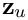where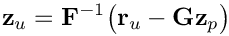. The values of a and b are given by: The values of dx and dy are given by: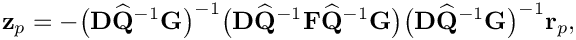and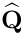Taken from: Saad Y."Iterative methods for sparse linear systems", p.193. We also check to see that sn is always a real (nonnegative) number. See pp.193-194 for an explanation.

Definition at line 1106 of file complex_smoother.h.

References oomph::IterativeLinearSolver::tolerance().

## ◆ iterations()

template<typename MATRIX >
 unsigned oomph::ComplexGMRES< MATRIX >::iterations ( ) const
inlinevirtual

Number of iterations taken.

Implements oomph::IterativeLinearSolver.

Definition at line 918 of file complex_smoother.h.

References oomph::ComplexGMRES< MATRIX >::Iterations.

## ◆ operator=()

template<typename MATRIX >
 void oomph::ComplexGMRES< MATRIX >::operator= ( const ComplexGMRES< MATRIX > & )
delete

Broken assignment operator.

## ◆ solve() [1/2]

template<typename MATRIX >
 void oomph::ComplexGMRES< MATRIX >::solve ( DoubleMatrixBase *const & matrix_pt, const Vector< double > & rhs, Vector< double > & result )
inlinevirtual

Linear-algebra-type solver: Takes pointer to a matrix and rhs vector and returns the solution of the linear system Call the broken base-class version. If you want this, please implement it.

Reimplemented from oomph::LinearSolver.

Definition at line 910 of file complex_smoother.h.

References oomph::LinearSolver::solve().

## ◆ solve() [2/2]

template<typename MATRIX >
 void oomph::ComplexGMRES< MATRIX >::solve ( Problem *const & problem_pt, DoubleVector & result )
inlinevirtual

Solver: Takes pointer to problem and returns the results vector which contains the solution of the linear system defined by the problem's fully assembled Jacobian and residual vector.

Implements oomph::LinearSolver.

Definition at line 892 of file complex_smoother.h.

## ◆ update()

template<typename MATRIX >
 void oomph::ComplexGMRES< MATRIX >::update ( const unsigned & k, const Vector< Vector< std::complex< double > > > & hessenberg, const Vector< std::complex< double > > & s, const Vector< Vector< DoubleVector > > & v, Vector< DoubleVector > & x )
inlineprivate

Helper function to update the result vector.

Definition at line 1029 of file complex_smoother.h.

References i, and s.

## ◆ Iterations

template<typename MATRIX >
 unsigned oomph::ComplexGMRES< MATRIX >::Iterations
private

Number of iterations taken.

Definition at line 1201 of file complex_smoother.h.

Referenced by oomph::ComplexGMRES< MATRIX >::iterations().

## ◆ Matrices_storage_pt

template<typename MATRIX >
 Vector oomph::ComplexGMRES< MATRIX >::Matrices_storage_pt
private

Vector of pointers to the real and imaginary part of the system matrix.

Definition at line 1204 of file complex_smoother.h.

## ◆ Matrix_can_be_deleted

template<typename MATRIX >
 bool oomph::ComplexGMRES< MATRIX >::Matrix_can_be_deleted
private

Boolean flag to indicate if the real and imaginary system matrices can be deleted.

Definition at line 1212 of file complex_smoother.h.

## ◆ Resolving

template<typename MATRIX >
 bool oomph::ComplexGMRES< MATRIX >::Resolving
private

Boolean flag to indicate if the solve is done in re-solve mode, bypassing setup of matrix and preconditioner.

Definition at line 1208 of file complex_smoother.h.

The documentation for this class was generated from the following file: